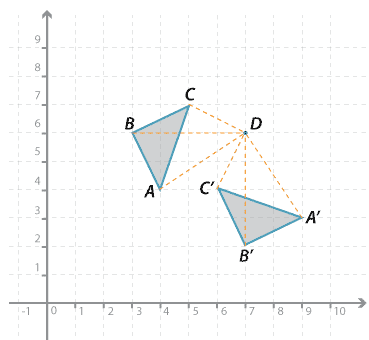### Rotations of multiples of 90 ° about a pointDetailed description

In the figure above, $$\triangle ABC$$ is rotated 90 ° in a clockwise direction about point $$D$$ to the image $$\triangle A^\prime B^\prime C^\prime$$.

The point $$A$$ in the diagram above is rotated 90 ° in a clockwise direction about point $$D$$. Note that $$AD = A^\prime D$$ and $$\angle AD A^\prime = 90^\circ$$.

Using coordinates we can describe the rotation of the vertices:

$$A(4, 4) \rightarrow A^\prime (9, 3); B(3, 6) \rightarrow B^\prime (7, 2); C(5, 7) \rightarrow C^\prime (6, 4)$$.